# Art gallery theorems

(diff) ← Older revision | Latest revision (diff) | Newer revision → (diff)
A collection of results similar to Chvátal's original theorem (cf. Chvátal theorem). Each specifies tight bounds on the number of guards of various types that can visually cover a polygonal region. A guardsees a pointif and only if there is an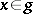such that the segment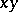is nowhere exterior to the closed polygon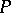. A polygon is covered by a set of guards if every point in the polygon is visible to some guard. Types of guards considered include point guards (any point), vertex guards (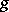is a vertex of), diagonal guards (is a nowhere exterior segment between vertices), and edge guards (is an edge of). An art gallery theorem establishes the exact bound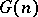for specific types of guards in a specific class of polygons, whereis the maximum over all polygonswithvertices, of the minimum number of guards that suffice to cover.
For simple polygons, the main bounds forare:vertex guards (the Chvátal theorem),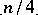diagonal guards [a6], andvertex guards for orthogonal polygons (polygons whose edges meet orthogonally) [a5]. No tight bound for edge guards has been established.
Attention can be turned to visibility outside the polygon: for coverage of the exterior of the polygon, one needspoint guards (any point not in the interior of) [a6]; for coverage of both interior and exterior, one needs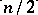vertex guards [a2]. For polygons with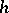holes and a total ofvertices, the main results are:point guards for simple polygons [a1], [a4], andpoint guards for orthogonal polygons (independent of) [a3]. Both hole problems remain open for vertex guards. See [a7] for a survey updating [a6].